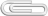Mathematic Applications

 BE5B15MAAMathematic ApplicationsExtent of Education: 0+4 Lecturers (garant): Kyncl J.Type of Subject: PEnd of Subject: Z, ZK Responsible Department: 13115 Credits: 4 Semester: L

Abstract:

The aim of the course is to obtain knowledge about mathematic programs used in electrical engineering. Student will acquire basic knowledge about MATLAB, MATHEMATICA and mathematical model assessment.

Seminar notes (Th 14:30 – 17:45, posl. T2:C3-132):

Week Description Attachments
1. Introduction into Wolfram Mathematica: basic syntax, help, variables, complex numbers, basic plotting and graph manipulations
2. Principles of procedural, functional and pattern-oriented programming
3. Functions in Mathematica: Blank, Set, SetDelayed, Module
4. Functions in Mathematica: piecewise functions, overloaded functions, tracing the evaluation of functions
5. Creation and manipulation of Lists, functions and Lists: Table, Part, Map, Apply
6. Using Nest and NestList for numerical algorithms, Euler and Runge-Kutta method
7. Examples of advanced use of Mathematica
8. MATLAB
9. MATLAB
10. MATLAB
11. MATLAB
14.

During the semester, there will be three tests (total max. 30 pts) from WM, without any minimal points restriction.

The exam will have two parts WM and Matlab (max. 40 points)

Students will be graded according to the ECTS Scale.

Literature:

1. Introduction to Wolfram Mathematica2. Downloading of student version of Wolfram Mathematica a Matlab3. Stephen Wolfram: An elementary introduction to the Wolfram Language4. Wolfram Mathematica### Přílohy

 CAO-RungeKuttaEng.nb44037 bytes Examples.zip639548 bytes Intro_WM.rar3136013 bytes MAA2018-1.nb100973 bytes dataTest.csv601 bytes dataUI.csv7294 bytes dataUI.xls14848 bytes datsTask2.csv23462 bytes englMAA2017-1.nb84828 bytes englMAA2017.nb69877 bytes exam04062019.zip3821 bytes exam06062017.nb6187 bytes examMaa.zip44102 bytes integration.nb4668 bytes lecture English2015.nb52955 bytes plTask2.png26346 bytes scope_7.csv631000 bytes sem1.nb89099 bytes seminar14032019.nb18646 bytes seminar1_21_02_19.nb715271 bytes seminar2132019.nb104228 bytes seminar2832019.nb117847 bytes seminar2_28_02_19.nb58696 bytes seminar3_07_03.nb186391 bytes test.csv635059 bytes test25042017Final.nb5588 bytes test3.nb5255 bytes